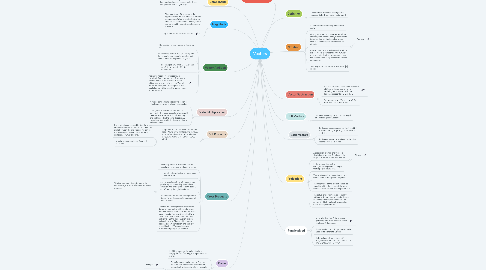# Vectors

Get Started. It's FreeVectors## 5. Dot Products

### 5.1. Dot products, u * v, between two vectors can be found using the following formula. If v = <v1,v2,v3> and u=<u1,u2,u3> then the dot product is u * v = u1*v1 + u2*v2 + u3*v3.

5.1.1. Dot products can be used to find the angle between two vectors. If we let theta be the angle between the two vectors we can use the following formula to solve for theta: cos(theta) = (u*v)/(||u||*||v||)

5.1.2. v and u are perpendicular if and only if u * v = 0

## 7. Cross Products

### 7.3. u x v is a vector with length (area of the parallelogram induced by u and v) and direction (the orthogonal to both u and v as defined by the right hand rule)

7.3.1. This is a picture of the right hand rule. In this picture, b is the direction of the cross product.

8.2.1. Example

10.2.1. Example

14.1.1. Picture